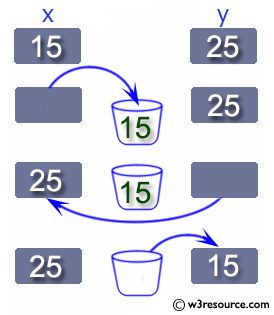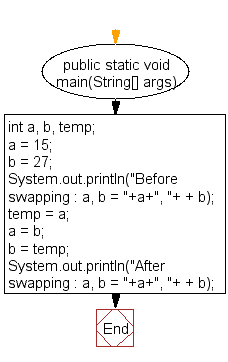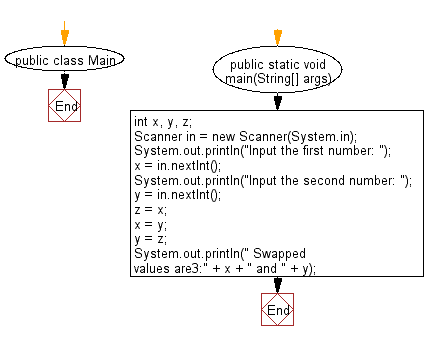﻿ Java exercises: Swap two variables - w3resource# Java Exercises: Swap two variables

## Java Basic: Exercise-15 with Solution

Write a Java program to swap two variables.

Java: Swapping two variables

Swapping two variables refers to mutually exchanging the values of the variables. Generally, this is done with the data in memory.

The simplest method to swap two variables is to use a third temporary variable :

```define swap(a, b)
temp := a
a := b
b := temp
```

Pictorial Presentation:Sample Solution:

Java Code:

``````public class Exercise15 {

public static void main(String[] args) {

int a, b, temp;
a = 15;
b = 27;
System.out.println("Before swapping : a, b = "+a+", "+ + b);
temp = a;
a = b;
b = temp;
System.out.println("After swapping : a, b = "+a+", "+ + b);
}
}
```
```

Without using third variable.

Sample Solution:-

Java Code:

``````public class Exercise15 {
public static void main(String[] args) {
// int, double, float
int a, b;
a = 15;
b = 27;
System.out.println("Before swapping : a, b = "+a+", "+ + b);
a = a + b;
b = a - b;
a = a - b;
System.out.println("After swapping : a, b = "+a+", "+ + b);
}

}
```
```

Sample Output:

```Before swapping : a, b = 15, 27
After swapping : a, b = 27, 15
```

Flowchart:Sample Solution (Input from the user):

Java Code:

``````import java.util.Scanner;
public class Main {
public static void main(String[] args) {
int x, y, z;
Scanner in = new Scanner(System.in);

System.out.println("Input the first number: ");
x = in.nextInt();
System.out.println("Input the second number: ");
y = in.nextInt();

z = x;
x = y;
y = z;

System.out.println(" Swapped values are3:" + x + " and " + y);
}
}
```
```

Sample Output:

```Input the first number:
36
Input the second number:
44
Swapped values are:44 and 36
```

Flowchart:Java Code Editor:

What is the difficulty level of this exercise?

Test your Programming skills with w3resource's quiz.

﻿

## Java: Tips of the Day

countOccurrences

Counts the occurrences of a value in an array.

Use Arrays.stream().filter().count() to count total number of values that equals the specified value.

```public static long countOccurrences(int[] numbers, int value) {
return Arrays.stream(numbers)
.filter(number -> number == value)
.count();
}
```

Ref: https://bit.ly/3kCAgLb